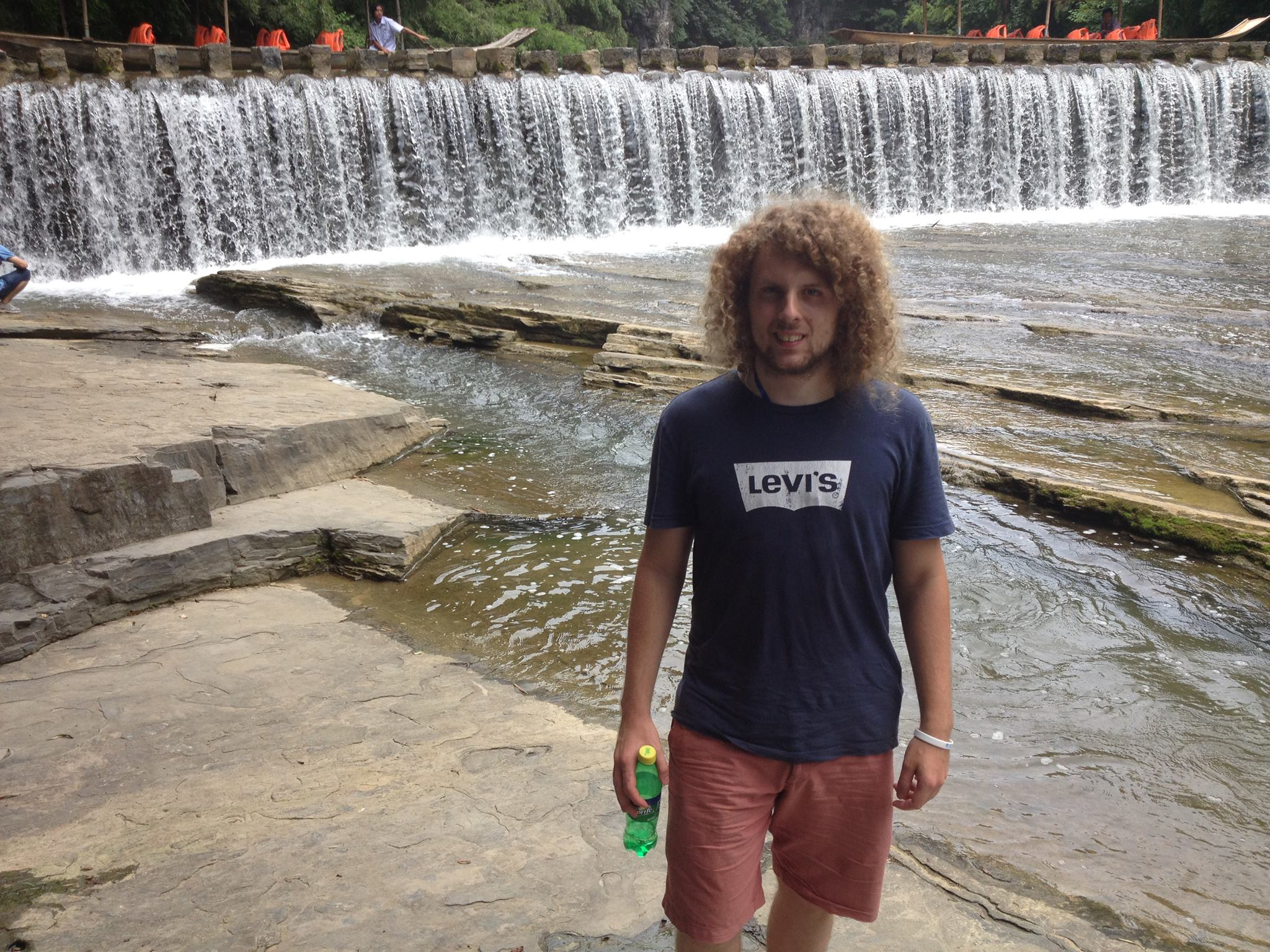# William Hardesty## Contact Information

Email: hardes11 at gmail dot com

I am a mathematician, working in geometric representation theory, with an emphasis on the modular representation theory of reductive algebraic groups, and in related areas of geometry and combinatorics. I have been an Assistant Professor of Mathematics at Westlake University, a Research Fellow in the School of Mathematics and Statistics at the University of Sydney, and a Postdoctoral Researcher at Louisiana State University. I recieved my Ph.D. from the University of Georgia in 2016 under the supervision of Daniel Nakano.

## Research

### Preprints

1. On the centralizer of a balanced nilpotent section.
Preprint arXiv:1810.06157, 21 pp. (submitted)

### Published/Accepted

1. (with P. Achar) Silting complexes of coherent sheaves and the Humphreys conjecture.
To appear in Duke Mathematical Journal, 32 pp.

2. (with P. Achar) Co-t-structures on derived categories of coherent sheaves and the cohomology of tilting modules.
To appear in Representation Theory of the American Mathematical Society, 35 pp.

3. (with P. Achar) Nilpotent centralizers and good filtrations.
Transformation Groups (2022).

4. (with P. Achar, S. Riche) Integral exotic sheaves and the modular Lusztig-Vogan bijection.
J. London Math. Soc. 106 (2022), 2403-2458.

5. (with P. Achar, S. Riche) Representation theory of disconnected reductive groups.
Documenta Mathematica, (2020), 25, 2149-2177.

6. Explicit calculations in an infinitesimal singular block of $SL_n$.
Proceedings of the Edinburgh Mathematical Society, 65(1), (2022), 19-52.

7. (with P. Achar, S. Riche) Conjectures on tilting modules and antispherical $p$-cells.
To appear in RIMS Kôkyûroku Bessatsu, 20 pp.

8. (with P. Achar) Calculations with graded perverse-coherent sheaves.
The Quarterly Journal of Mathematics, Volume 70, Issue 4 (2019) 1327-1352.

9. (with P. Achar, S. Riche) On the Humphreys conjecture on support varieties of tilting modules.
Transformation Groups 24, 597-657 (2019).

10. On support varieties and the Humphreys conjecture in type $A$.

11. (with D. Nakano, P. Sobaje) On the Existence of Mock Injective Modules for Algebraic Groups.
Bull. Lond. Math. Soc. 49 (2017), 806-817.

12. Support varieties of line bundle cohomology groups for $G=SL_3(k)$.
J. Algebra 448 (2016), 127-173.

## Teaching

• MATH 2065: Ordinary Differential Equations (Spring 2018, LSU)
• MATH 1551: Honors Calculus I (Fall 2016, LSU)
• MATH 2250: Calculus I (Fall 2015, UGA)
• MATH 1113: Pre-Calculus (Fall 2014, UGA)
• MATH 2250: Calculus I (Spring 2014, UGA)
• MATH 1113: Pre-Calculus (Fall 2013, UGA)